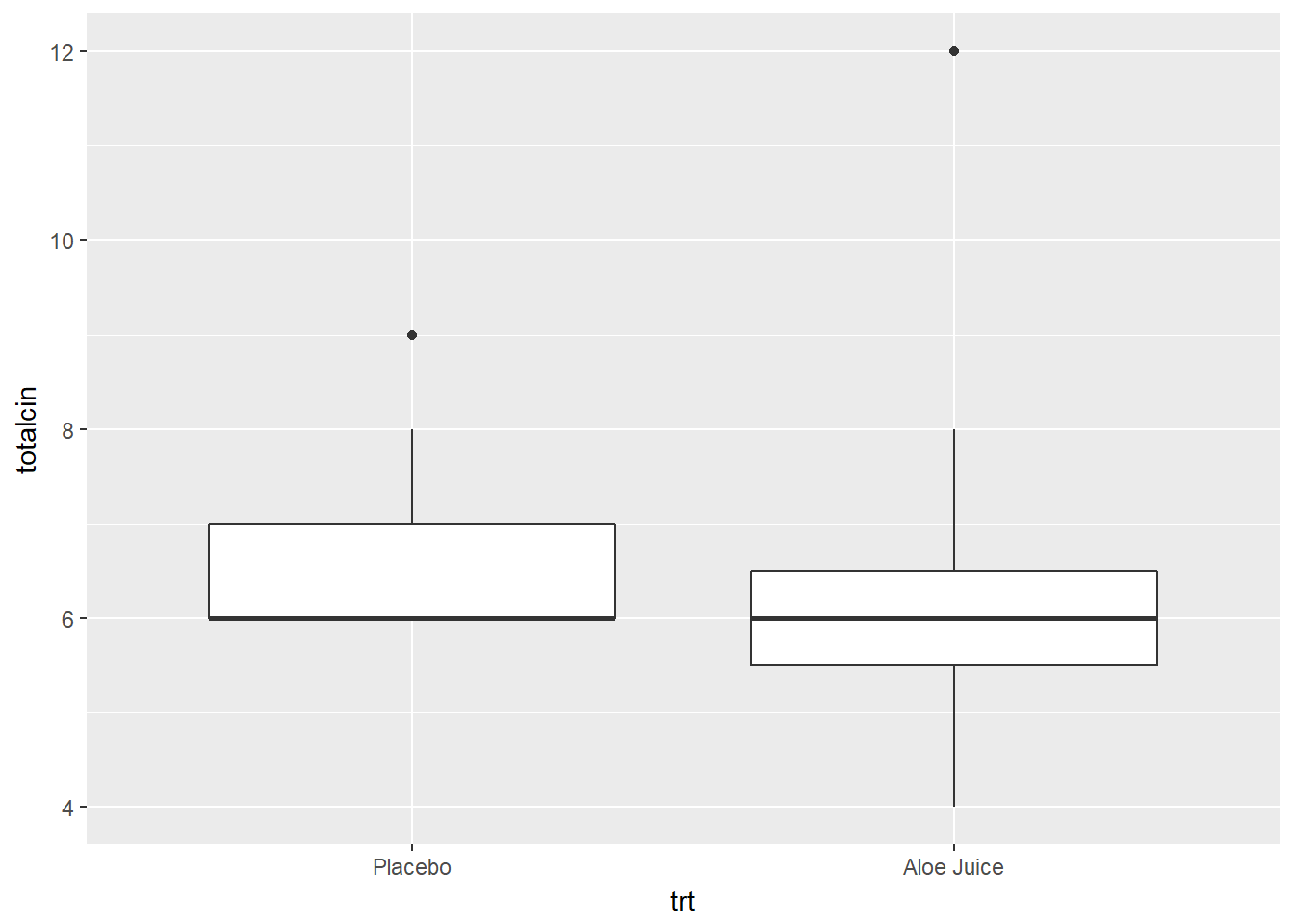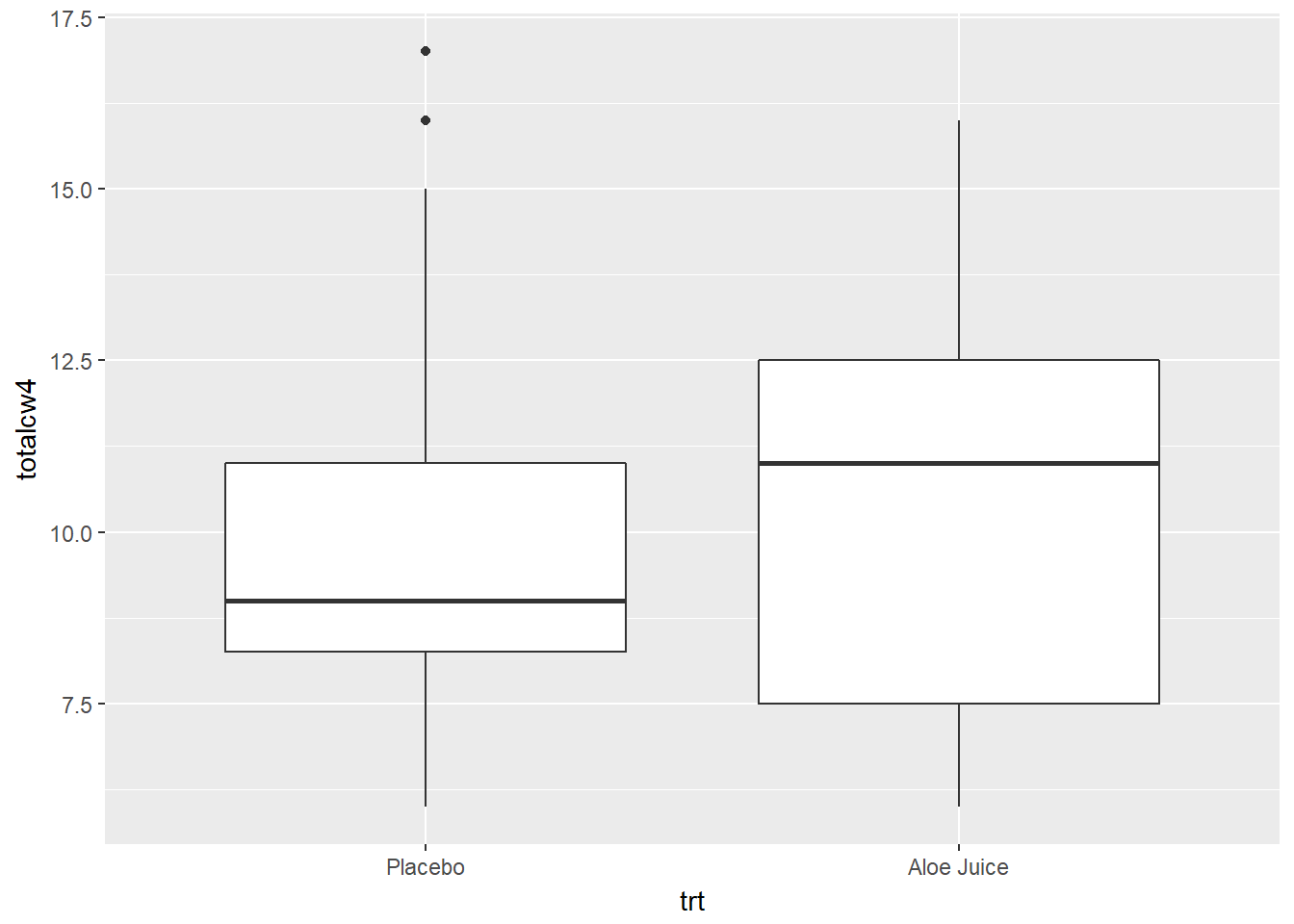# Chapter 7 t TEST FOR THE DIFFERENCE IN 2 MEANS, INDEPENDENT SAMPLES

Required Packages

library(tidyverse)    # Loads several very helpful 'tidy' packages
library(haven)        # Read in SPSS datasets
library(car)          # Companion for Applied Regression (and ANOVA)

Example: Cancer Experiment

The Cancer dataset was introduced in chapter 3.

Check Means and SD’s

cancer_clean %>%
dplyr::group_by(trt) %>%
furniture::table1(totalcin, totalcw4)

--------------------------------
trt
Placebo    Aloe Juice
n = 14     n = 11
totalcin
6.6 (0.9)  6.5 (2.1)
totalcw4
10.1 (3.6) 10.6 (3.5)
--------------------------------

## 7.1 Assumtion Check: Eyeball method

Do the two groups, treatment and control, have the same amount of spread (standard deviations) BUT different centers (means)?

cancer_clean %>%
ggplot(aes(x = trt,
y = totalcin)) +
geom_boxplot()cancer_clean %>%
ggplot(aes(x = trt,
y = totalcw4)) +
geom_boxplot()## 7.2 Assumtion Check: Homogeneity of Variance

Before performing the $$t$$ test, check to see if the assumption of homogeneity of variance is met using Levene’s Test. For a independent samples t-test for means, the groups need to have the same amount of spread (SD) in the measure of interest.

Use the car:leveneTest() function to do this. Inside the funtion you need to specify at least three things (sepearated by commas):

• the formula: continuous_var ~ grouping_var (replace with your variable names)
• the dataset: data = . to pipe it from above
• the center: center = "mean" since we are comparing means

Do the participants in the treatment and control groups have the same spread in oral condition at BASELINE?

cancer_clean %>%
car::leveneTest(totalcin ~ trt,    # formula: continuous_var ~ grouping_var
data = .,          # pipe in the dataset
center = "mean")   # The default is "median"
Levene's Test for Homogeneity of Variance (center = "mean")
Df F value Pr(>F)
group  1  2.2103 0.1507
23               

No violations of homogeneity were detected, $$F(1, 23) = 2.210, p = .151$$.

Do the participants in the treatment and control groups have the same spread in oral condition at the FOURTH WEEK?

cancer_clean %>%
car::leveneTest(totalcw4 ~ trt,    # formula: continuous_var ~ grouping_var
data = .,          # pipe in the dataset
center = "mean")   # The default is "median"
Levene's Test for Homogeneity of Variance (center = "mean")
Df F value Pr(>F)
group  1       0  0.995
23               

No violations of homogeneity were detected, $$F(1, 23) = 0, p = .995$$.

## 7.3 2 independent Sample Means

Use the same t.test() funtion we have used in the prior chapters. This time you need to speficy a few more options.

• the formula: continuous_var ~ grouping_var (replace with your variable names)

• the dataset: data = . to pipe it from above

• is homogeneity satified?: var.equal = TRUE (NOT the default)

• number of tails: alternative = "two.sided"

• independent vs. paired: paired = FALSE

• confidence level: conf.level = #

Do the participants in the treatment group have a different average oral condition at BASELINE, compared to the control group?

# Minimal syntax
cancer_clean %>%
t.test(totalcin ~ trt,   # formula: continuous_var ~ grouping_var
data = .,         # pipe in the dataset
var.equal = TRUE) # HOV was violated (option = TRUE)

Two Sample t-test

data:  totalcin by trt
t = 0.18566, df = 23, p-value = 0.8543
alternative hypothesis: true difference in means is not equal to 0
95 percent confidence interval:
-1.185479  1.419245
sample estimates:
mean in group Placebo mean in group Aloe Juice
6.571429                 6.454545 

No evidence of a differnece in mean oral condition at baseline, $$t(23) = 0.186, p = .854$$.

Do the participants in the treatment group have a different average oral condition at the FOURTH WEEK, compared to the control group?

# Fully specified function
cancer_clean %>%
t.test(totalcw4 ~ trt,             # formula: continuous_var ~ grouping_var
data = .,                   # pipe in the dataset
var.equal = TRUE,           # default: HOV was violated (option = TRUE)
alternative = "two.sided",  # default: 2 sided (options = "less", "greater")
paired = FALSE,             # default: independent (option = TRUE)
conf.level = .95)           # default: 95% (option = .9, .90, ect.)

Two Sample t-test

data:  totalcw4 by trt
t = -0.34598, df = 23, p-value = 0.7325
alternative hypothesis: true difference in means is not equal to 0
95 percent confidence interval:
-3.444215  2.457202
sample estimates:
mean in group Placebo mean in group Aloe Juice
10.14286                 10.63636 

No evidence of a differnece in mean oral condition at the fourth week, $$t(23) = -0.350, p = .733$$.